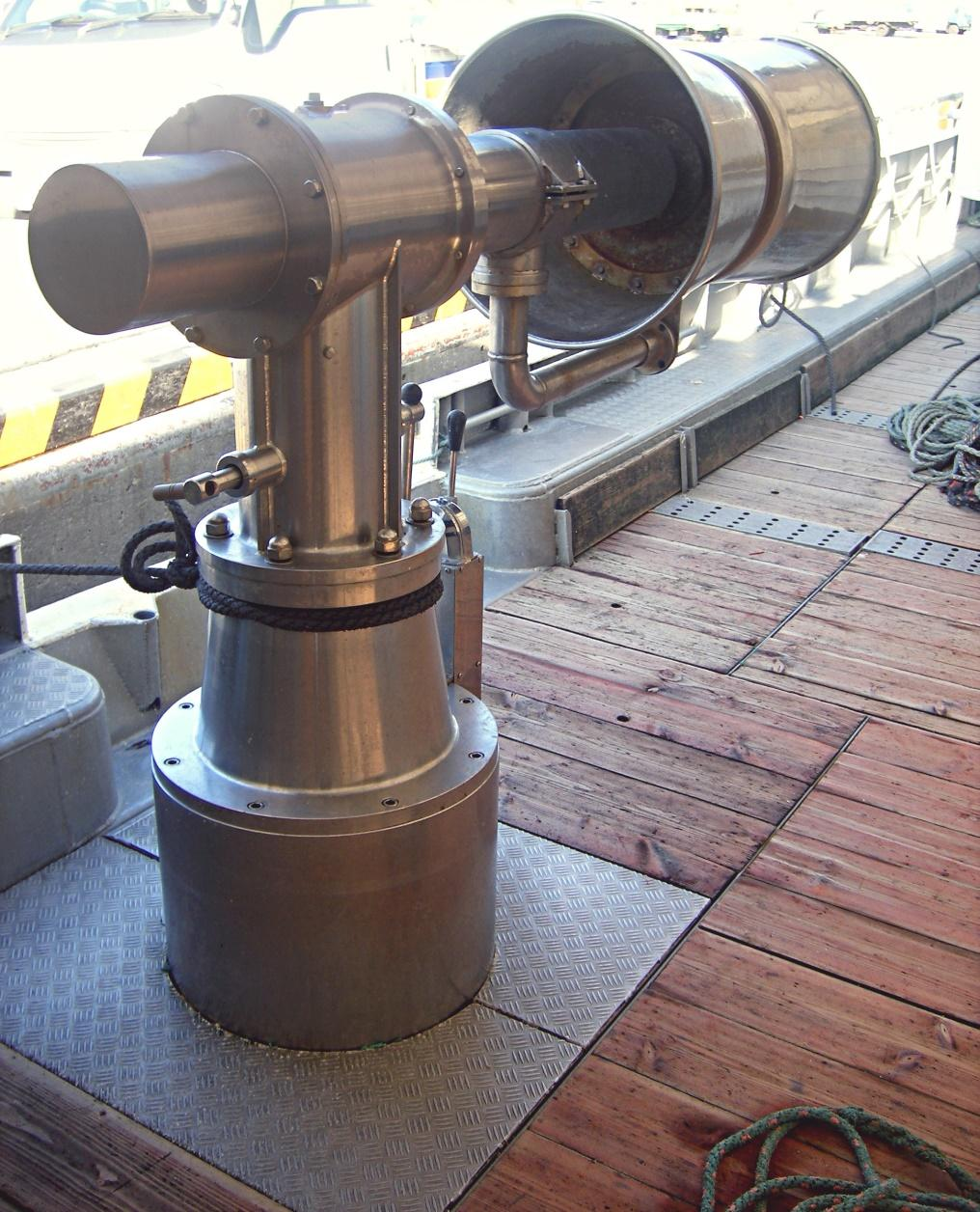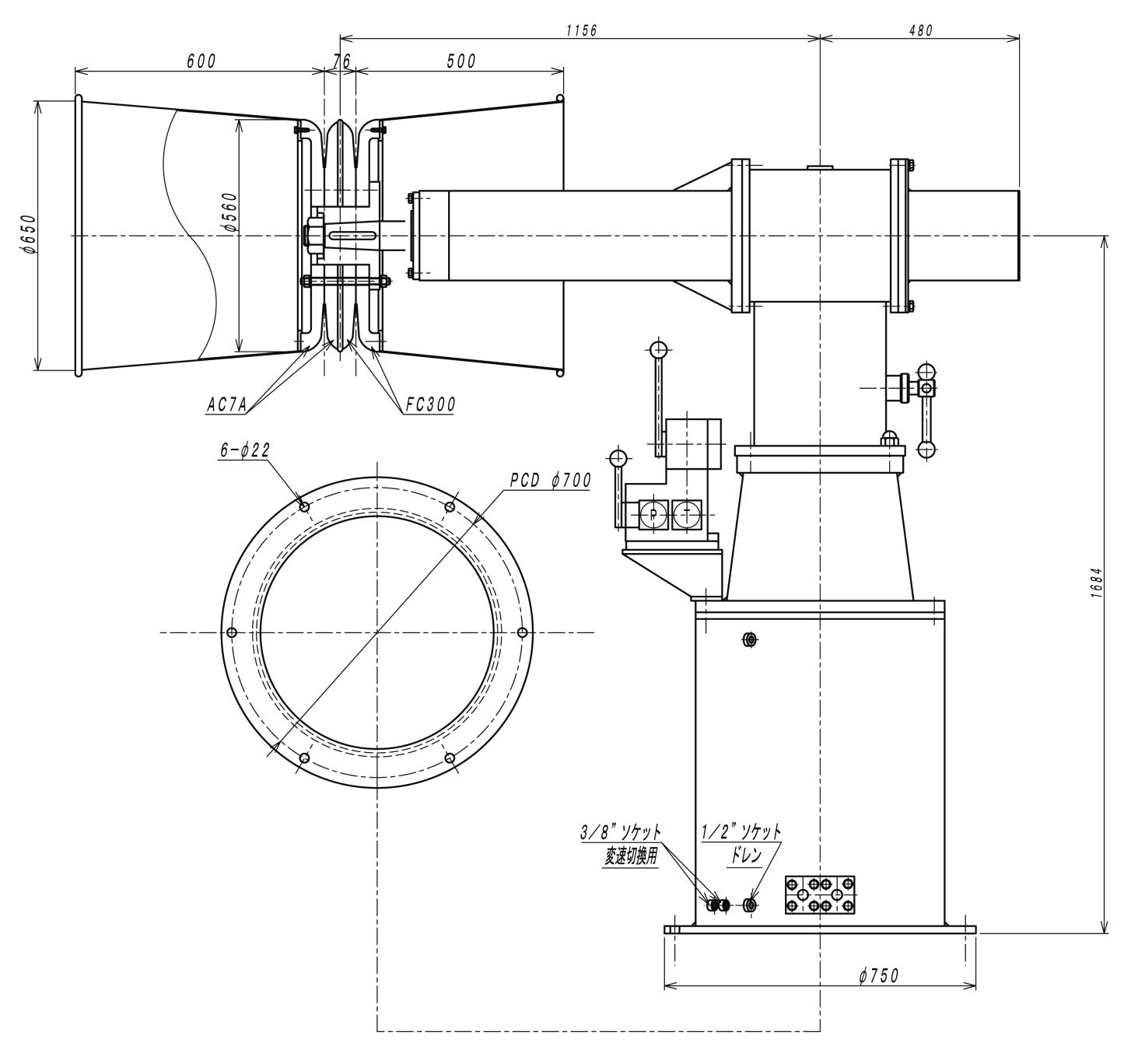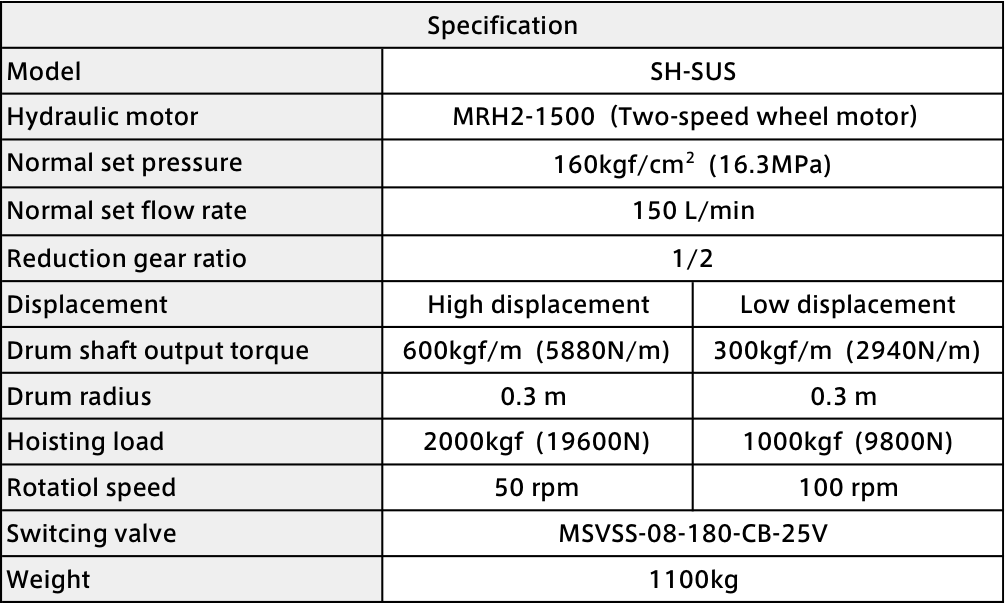# SUS Steel Net Hauler## SUS Steel Net Hauler

It is good for the net hoisting on board.
It contributes to the saving energy of the net hoisting work and high volume of landing.

Hoisting speed with two speed motors can be provided.
Various drums can be provided in response to the application.

### Outer dimension### Specification

Ex) Model : GH218## Main calculation formula of hydraulic pressure device

1. Hydraulic pump
* 1) axis input of a pump
Ls= (P・Q/60 η) ・ 102.* 2) Oil power of the pump
Lp= P・Q/60= η ・ Ls ・ 10-2* 3) Total efficiency of the pump
η = η v ・ηt ・ 10-2* 4) Capacity efficiency of the pump
ηv= (Q/Qth)・ 100≒ (Q/Q0)・ 100.* 5) Efficiency of the motor
η e = Ls/Le
2. Hydraulic motor
* 1)Theoretical Displacement volume of Hydraulic motor
Dth= (2 π・ T)/(P・ η t) ・ 102.* 2) Output power of Hydraulic motor
Ls=2π・ T・N/60000= η ・(PQ/60) ・ 102.* 3) Input power of Hydraulic motor
Lm=PQ・60* 4) Capacity efficiency of Hydraulic motor
η (v= DthN/Q)・ 10-1* 5) Torque efficiency of Hydraulic motor
η t= (2 π ・ T/PDth) ・ 102.* 6) Total efficiency of Hydraulic motor
η = η・ v ・η t・ 102= (Ls/Lm)・ 102= (2 π  TN/PQ)・ 10-1.
3. Cylinder
* 1) Necessary pressure to a cylinder
P1= (1/ A1) (F/ η c+P2 A2102) and 10-2* 2) Necessary flow rate for a cylinder
Q= A1 v 10-1+QL* 3) Driving force of a cylinder
Acceleration ability
F1=m・α =mv1/t.
Static wear resistance
F2=  μ s・mg.
Dynamic wear resistance
F3=  μ・ dm・g.

### The explanations of symbols

Ls: input of pump shaft, output power of the motor, the output power of the motor (kW)
Lp: oil power of the pump (kW)
Le: input power of the motor (kW)
Lm: input power of the motor (kW)
P: Discharge pressure of pump, differential-pressure of the input/output port of a motor (MPa)
P1: necessary pressure to cylinder (MPa)
P2: pressure of the cylinder inflow (MPa)
Q: discharge amount at the time of discharge pressure P,
inflow oil amount to the motor, necessary flow rate of the cylinder (large/min)
Qth: theoretical discharge amount (l/min)
Qo: discharge amount at the time of discharge pressure P≒0, (l/min)
QL: Leak inside the cylinder (l/min)
T: shaft torque (N・m)
N: 　number of rotations (min-1)
η: Total efficiency of the pump, total efficiency of the motor (the %)
η v: capacity efficiency of pump, capacity efficiency of the motor (the %)
η t: torque efficiency of pump, torque efficiency of the motor (the %)
η e: efficiency of motor (the %)
η c: driving force efficiency of cylinder (0.9-0.95)
Dth: theoretical displacement volume of the motor (cm3/rev)
A1: inlet side pressure receiving area of cylinder (cm2)
A2: outlet side pressure receiving area of cylinder(cm2)
F: cylinder driving force (N)
F1: cylinder acceleration force (N)
F2: static wear resistance (N)
F3: dynamic wear resistance (N)
v: The speed of the cylinder (m/min)
v1: The speed after acceleration (m/s)
m: The mass of the load (KG)
α: acceleration (m/s2)
t: acceleration time (s)
μ s: static friction coefficient
μ d: dynamic friction coefficient
g: acceleration of the gravity (m/s2)